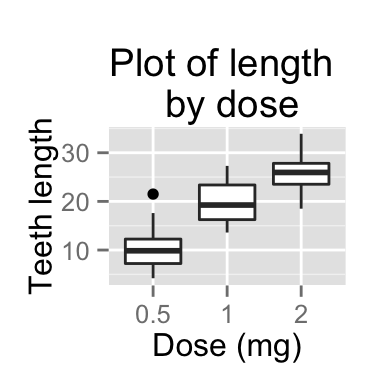# ggplot2 title : main, axis and legend titles

The aim of this tutorial is to describe how to modify plot titles (main title, axis labels and legend titles) using R software and ggplot2 package.

The functions below can be used :

``````ggtitle(label) # for the main title
xlab(label) # for the x axis label
ylab(label) # for the y axis label
labs(...) # for the main title, axis labels and legend titles``````

The argument label is the text to be used for the main title or for the axis labels.

# Prepare the data

ToothGrowth data is used in the following examples.

``````# convert dose column from a numeric to a factor variable
ToothGrowth\$dose <- as.factor(ToothGrowth\$dose)
``````##    len supp dose
## 1  4.2   VC  0.5
## 2 11.5   VC  0.5
## 3  7.3   VC  0.5
## 4  5.8   VC  0.5
## 5  6.4   VC  0.5
## 6 10.0   VC  0.5``````

Make sure that the variable dose is converted as a factor using the above R script.

# Example of plot

``````library(ggplot2)
p <- ggplot(ToothGrowth, aes(x=dose, y=len)) + geom_boxplot()
p``````# Change the main title and axis labels

Change plot titles by using the functions ggtitle(), xlab() and ylab() :

``````p + ggtitle("Plot of length \n by dose") +
xlab("Dose (mg)") + ylab("Teeth length")``````Note that, you can use \n to split long title into multiple lines.

Change plot titles using the function labs() as follow :

``````p +labs(title="Plot of length \n by dose",
x ="Dose (mg)", y = "Teeth length")``````It is also possible to change legend titles using the function labs():

``````# Default plot
p <- ggplot(ToothGrowth, aes(x=dose, y=len, fill=dose))+
geom_boxplot()
p
# Modify legend titles
p + labs(fill = "Dose (mg)")``````# Change the appearance of the main title and axis labels

Main title and, x and y axis labels can be customized using the functions theme() and element_text() as follow :

``````# main title
p + theme(plot.title = element_text(family, face, colour, size))
# x axis title
p + theme(axis.title.x = element_text(family, face, colour, size))
# y axis title
p + theme(axis.title.y = element_text(family, face, colour, size))``````

The arguments below can be used for the function element_text() to change the appearance of the text :

• family : font family
• face : font face. Possible values are “plain”, “italic”, “bold” and “bold.italic”
• colour : text color
• size : text size in pts
• hjust : horizontal justification (in [0, 1])
• vjust : vertical justification (in [0, 1])
• lineheight : line height. In multi-line text, the lineheight argument is used to change the spacing between lines.
• color : an alias for colour

``````# Default plot
p <- ggplot(ToothGrowth, aes(x=dose, y=len)) + geom_boxplot() +
ggtitle("Plot of length \n by dose") +
xlab("Dose (mg)") + ylab("Teeth length")
p
# Change the color, the size and the face of
# the main title, x and y axis labels
p + theme(
plot.title = element_text(color="red", size=14, face="bold.italic"),
axis.title.x = element_text(color="blue", size=14, face="bold"),
axis.title.y = element_text(color="#993333", size=14, face="bold")
)``````# Remove x and y axis labels

It’s possible to hide the main title and axis labels using the function element_blank() as follow :

``````# Hide the main title and axis titles
p + theme(
plot.title = element_blank(),
axis.title.x = element_blank(),
axis.title.y = element_blank())``````# Infos

This analysis has been performed using R software (ver. 3.1.2) and ggplot2 (ver. )